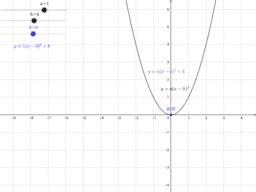•Manipulating the Graph of Sine

Activity

•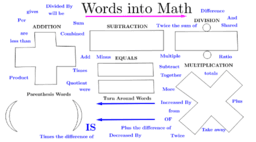Words into Math

Activity

•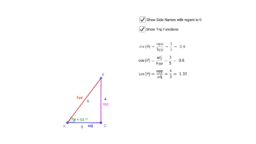Right Triangle Trig Calculator - Side control

Activity

•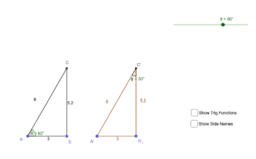Right Triangle Sine and Cosine Trig Investigation

Activity

•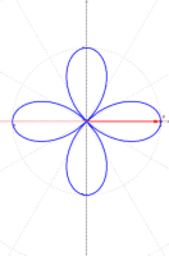polar example

Activity

•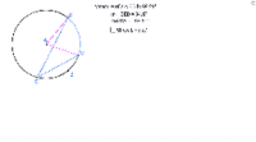Investigating Inscribed Angles

Activity

•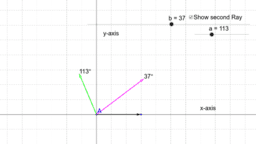Exploring Angle Measures

Activity

•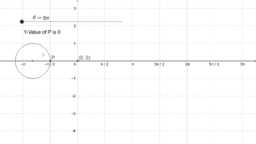Graph of Sine and the Unit Circle

Activity

•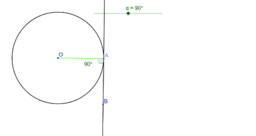Investigating lines that are tangent to circles.

Activity

•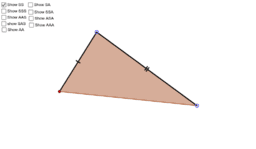Triangle Congruence

Activity

•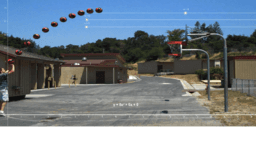When will the Basketball hit the hoop?

Activity

•Mr. Powers' Period 4 Random Student Picker

Activity

•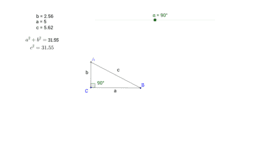Investigating the Pythagorean Theorem

Activity

•Intro to Polar Graphing

Activity

•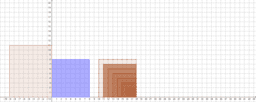Squares on a Checker Board

Activity

•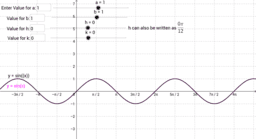Manipulating the Graph of Sine

Activity

•When will they meet. - Algebra word problem.

Activity

•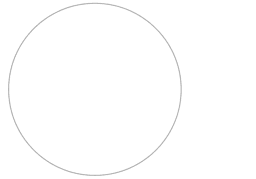Hyperbolic Geometry in the Poincaré Disk

Activity

•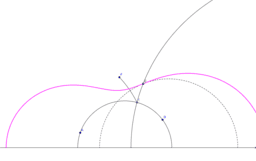Plotting the Parabola in the Hyperbolic Upper Half-Plane

Activity

•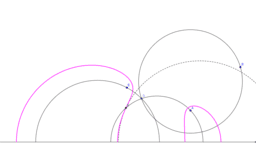Plotting Hyperbolas and Ellipses in Hyperbolic Half-Plane

Activity

•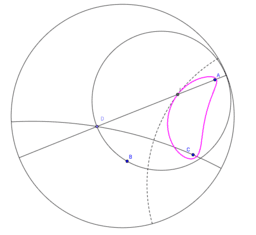Playing with the hyperbola and ellipse in the Poincaré disk

Activity

•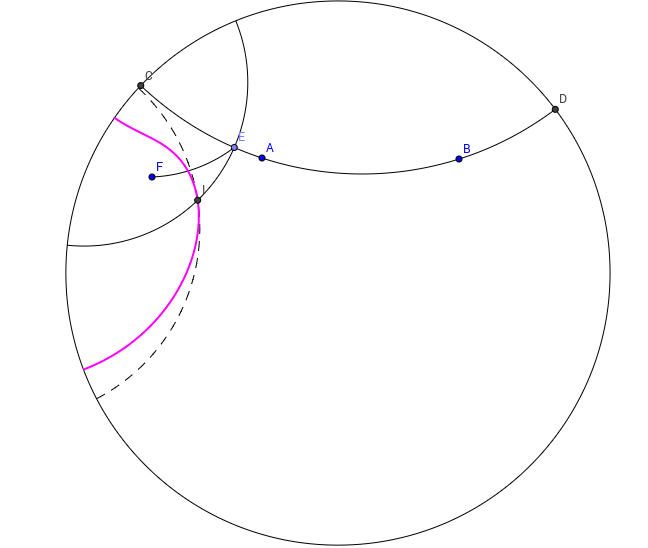Playing with the parabola in the Poincaré disk

Activity

•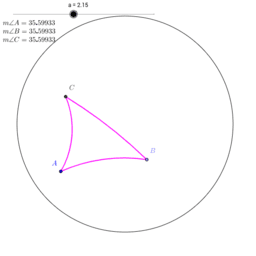Equilateral Triangles in the Poincare Disk

Activity

•